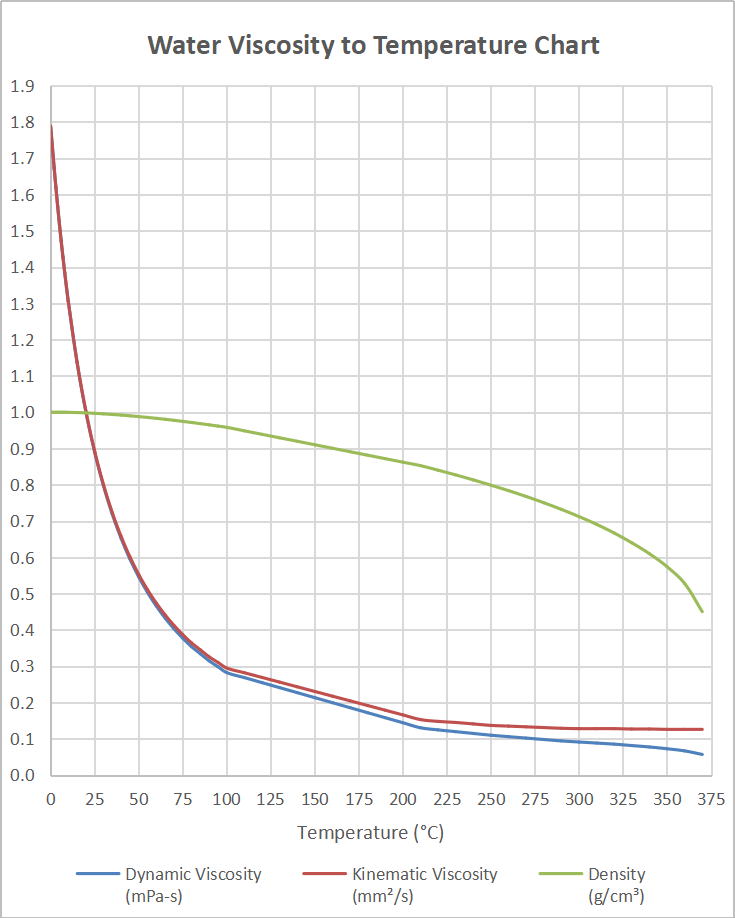# Water Viscosity Calculator

Created by Luis Hoyos
Last updated: Jul 28, 2022

Welcome to the water viscosity calculator, a tool that will let you calculate water viscosity (kinematic or dynamic viscosity) within the 0-370°C temperature range.

Although water viscosity in cp is 1.306 cP at 10°C (1 cP = mPa⋅s), it can vary enormously with temperature. For that reason, we created this calculator.

## Viscosity meaning

In simple words, viscosity means resistance to deformation. For the case of liquids, viscosity refers to how "thick" they are. For example, in the next pair of fluids, the fluid on the right has a greater viscosity:

Consider a fluid layer of constant thickness between two very large parallel plates (or two parallel plates immersed in a body of fluid), as in the following image:

While the lower plate remains stationary, we apply a force on the upper plate over an area, causing shear stress:

𝜏 = F/A

where:

• 𝜏 — Shear stress;
• F — Applied force; and
• A — Area of the force application.

After reaching stable conditions, the upper plate and the fluid portion in contact with it start to move at a constant speed. This speed varies along the thickness, going down to zero in the stationary plate (something known as the non-slip condition), and this variation creates a velocity gradient. It turns out to be that the shear stress is proportional to this velocity gradient, being viscosity the constant of proportionality:

𝜏 = μ(du/dy)

where:

• μ — Dynamic viscosity;
• u — Velocity of some layer of fluid;

Therefore, we can say that the greater the viscosity, the greater the force we'll need to apply to deform the fluid (or the greater its resistance to deformation).

## Dynamic vs. kinematic viscosity

When we say "viscosity", we're probably referring to dynamic viscosity (also known as absolute viscosity). In fluid mechanics, dynamic viscosity to density ratio frequently appears in equations. For that reason, we define a new property called viscosity, which consists of that ratio:

ν = μ/ρ

where:

• ν — Kinematic viscosity;
• μ — Dynamic viscosity; and
• ρ — Fluid Density.

## How does this water viscosity calculator work?

To calculate water viscosity, this calculator relies on experimental data. The graph below shows this data, displaying the dynamic viscosity of water in cP (or mPa⋅s), the kinematic viscosity in mm2/s, and the density in g/cm3.Water viscosity-temperature chart that displays the dynamic and kinematic viscosities as well as the density of water at temperatures ranging from 0°C to 370°C

As you can see, water viscosity decreases as temperature increases, and the relationship is stronger for lower temperatures.

Luis Hoyos
Temperature of water
°F
Dynamic viscosity, η
1.0016
mPa
⋅s
Kinematic viscosity, ν
0.0000108
ft²
/s
Density
62.32
lb/cu ft
People also viewed…

### Pump horsepower

Use this pump horsepower calculator to determine any pump's hydraulic and shaft power.